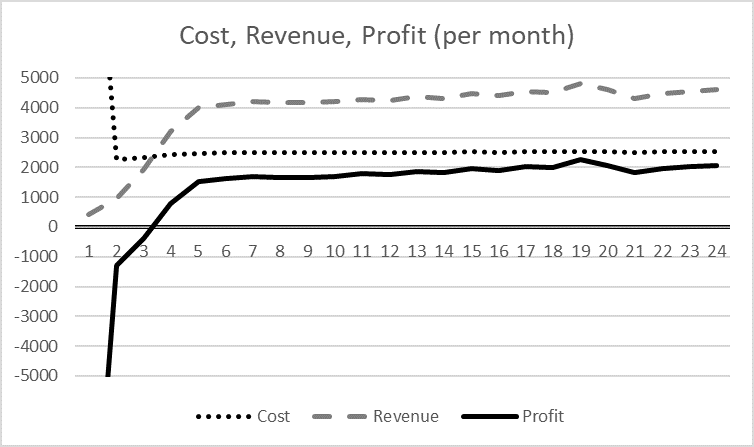Processing time: 90 minutes

With the last two tasks, you’ve laid the foundation and worked out the necessary figures to make an initial calculation of the financial potential of your business idea. So, today it remains mathematical for now!

Calculate in a spreadsheet (e.g., Excel, Google Sheets) the sum of fixed and variable costs, summarized by period, such as all one-off costs, all monthly costs, all annual costs, and all costs per customer. You can use this Excel example and adapt it to your needs.The figures can then be used to calculate the so-called break-even point, i.e., the point at which all your previous costs are covered, so that you start earning money from that point on (cf. Figure 123). In the yoga example, it takes twelve months until all initial and running costs are covered, and the first dollar is earned. It’s not uncommon for it to take one or two years to make a profit, depending on how high the fixed costs of a business idea are.Figure 12: The course of costs, revenues, and the result in the example

Now you can also estimate pre-tax income given your assumptions are confirmed. Compare Figure 134.Figure 13: Result per month in the example

The monthly income before taxes in this example is around \$2,000/month. Now you need to check whether this matches your expectations and needs, which you determined on Day 27. If not, you’ll either have to adjust your business idea so that the income is sufficient or check whether you can get by with less.

You must have a thorough understanding of the cost/revenue relationship. Now check out what happens when the various parameters change.

### Second part of the task:

Play around with the numbers a little to see how the result changes when the various influencing factors change:

• What if I only win half as many customers or 50% more than estimated?
• How does the revenue change if I increase or decrease the price of my service by 10%?
• What influence do the fixed costs have, i.e., how does the break-even point shift if I increase or reduce them by 10%, 25%, or 50%?
• What happens if I can convert fixed costs into variable costs (e.g., one-off acquisition costs through rent/leasing spread over the term; freelancers I only pay for hours worked or salaried employees with a fixed income)?

Write down the findings in your notebook – and, if necessary – on the Post-its in the Extended Canvas.

Wow! You’ve done quite a lot in the last three days, and for the first time, you’ve got a feeling for the financial potential of your idea. How does that feel?